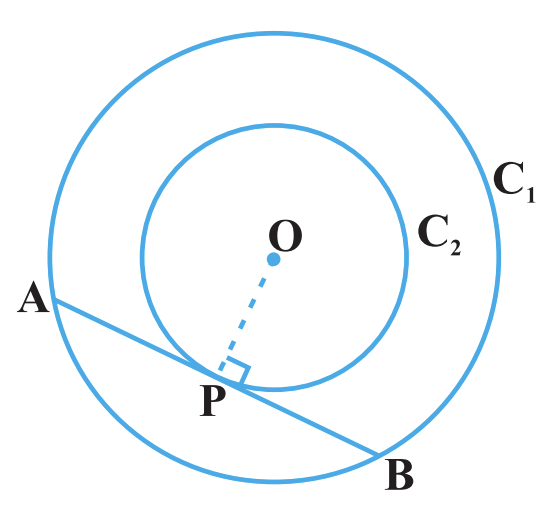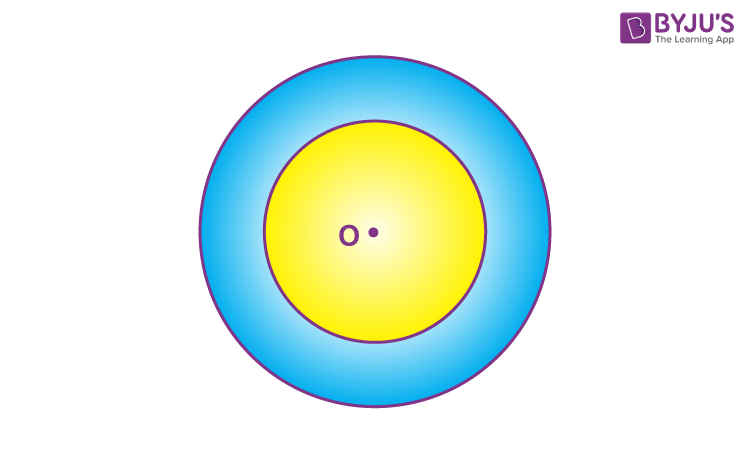# Concentric Circles

In Geometry, the objects are said to be concentric when they share a common centre. Circles, spheres, regular polyhedra, regular polygons are concentric as they share the same centre point. In Euclidean Geometry, two concentric circles should have different radii from each other. In this article, you will learn what are concentric circles, the theorem on concentric circles, the region between the concentric circles, equations, and examples in detail.

## Concentric Circles MeaningThe circles with a common centre are known as concentric circles and have different radii. In other words, it is defined as two or more circles that have the same centre point. The region between two concentric circles are of different radii is known as an annulus.

## Concentric Circle Equations

Let the equation of the circle with centre (-g, -f) and radius √[g2+f2-c] be

x2 + y2 + 2gx + 2fy + c =0

Therefore, the equation of the circle concentric with the other circle be

x2 + y2 + 2gx + 2fy + c’ =0

It is observed that both the equations have the same centre (-g, -f), but they have different radii, where c≠ c’

Similarly, a circle with centre (h, k), and the radius is equal to r, then the equation becomes

( x – h )2 + ( y – k )2 = r2

Therefore, the equation of a circle concentric with the circle is

( x – h )2 + ( y – k )2 = r12

Where r ≠ r1

By assigning different values to the radius in the above equation, we shall get a family of circles.

Concentric Circles – Theorem

In two concentric circles, the chord of the larger circle, which touches the smaller circle, is bisected at the point of contact.

Proof

Given:

Consider two concentric circles C1 and C2, with centre O and a chord AB of the larger circle C1, touching the smaller circle C2 at the point P as shown in the figure below.

Construction:

Join OP.To prove: AP = BP

Proof:

Since AB is the chord of larger circle C1, it becomes the tangent to C2 at P.

OP is the radius of circle C2.

We know that the radius is perpendicular to the tangent at the point of contact.

So, OP ⊥ AB

Now AB is a chord of the circle C1 and OP ⊥ AB.

Therefore, OP is the bisector of the chord AB.

Thus, the perpendicular from the centre bisects the chord, i.e., AP = BP.

### Region Between Concentric Circles

As mentioned above, the region between two concentric circles is called the annulus. However, we can find the perimeter and area of the annulus using appropriate formulas. The area of the annulus is calculated by subtracting the area of smaller circles from the area of the larger circle.Suppose R is the radius of the larger circle and r is the radius of the smaller circle such that the area of the region bounded by these two circles is given by:

Area of annulus = πR2 – πr2

### Concentric Circle Examples

Question: Find the equation of the circle concentric with the circle x2 + y2 + 4x – 8y – 6 =0, having the radius double of its radius.

Solution:

Given, circle equation: x2 + y2 + 4x – 8y – 6 =0

We know that the equation of the circle is x2 + y2 + 2gx + 2fy + c =0

From the given equation, the center point is (-2, 4)

Therefore, the radius of the given equation will be

r = √[g2+f2-c]

r = √[4+16+6]

r = √26

Let R be the radius of the concentric circle.

It is given that, the radius of the concentric circle is double of its radius, then

R = 2r

R = 2√26

Therefore, the equation of the concentric circle with the radius R and the center point (-g, -f ) is

( x – g )2 + ( y – f )2 = R2

(x + 2)2 + ( y – 4 )2 = (2√26 )2

x2 + 4x + 4 + y2 – 8y + 16 = 4 (26)

x2 + y2 + 4x – 8y +20 = 104

x2 + y2 + 4x – 8y – 84 = 0

Question 2:

Find the area between two concentric circles whose diameters are 35 cm and 21 cm.

Solution:

Given,

The diameters of the two circles are 35 cm and 21 cm.

So, R = 35/2 cm

r = 21/2 cm

Area of between concentric circles = πR2 – πr2

= (22/7) × (35/2) × (35/2) – (22/2) × (21/2) × (21/2)

= (22/7)[(35/2) × (35/2) – (21/2) × (21/2)]

= (22/7) × [(352 – 212)/4]

= (22/7) × (56 × 14)/4

= 616 cm2

## Video Lessons on Circles

### Introduction to Circles### Parts of a Circle### Area of a CircleStay tuned with BYJU’S – The Learning App to learn Maths-related concepts, and also watch engaging videos to learn with ease.

 Related Links Circles Radius of a Circle Properties of Circle Chord of a Circle

## Frequently Asked Questions on Concentric Circles

### What is meant by concentric circles?

The circles are said to be concentric circles, if they have the same center but different radii.

### What is the formula for concentric circles?

As we know, the equation of a circle with center (h, k) and radius “r” is: ( x – h)2 + ( y- k)2 = r2
Since the concentric circles are the circles with the same center, but different radius, say r1.
Thus, the formula for the concentric circle is given as:
( x – h)2 + ( y- k)2 = r12, such that r should not be equal to r1.

### How to find the area between two concentric circles?

Step 1: Find the area of the outer circle with radius r1.
Step 2: Find the area of the inner circle with radius r2.
Step 3: Subtract the inner circle area from the outer circle area.

### What is meant by annulus?

The region/area created between two concentric circles is called annulus.

### Does the concentric circle always have the same center?

Yes, the concentric circles always have the same center.

### Give an example of concentric circles.

An example of a concentric circle in real life is the wheel of a ship containing two concentric circles.

### What is the formula to find the area between two concentric circles?

If r1 is the radius of the outer circle and r2 is the radius of the inner circle, then the formula to calculate the area between two concentric circles is given by:
Area between two concentric circles = πr12 – πr22 or π (r12 – r22)

### Does the concentric circle have the same radii?

No, concentric circles have different radii.